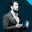visit

# Beyond CUDA: GPU Accelerated Python on Cross-Vendor Graphics Cards with Vulkan Kompute

This talk will provide practical insights on high performance GPU computing in Python using the Vulkan Kompute framework. We will cover the trends in GPU processing, the architecture of Vulkan Kompute, we will implement a simple parallel multiplication example, and we will then dive into a machine learning example building a logistic regression model from scratch which will run in the GPU.

In more detail these are the topics of the talk:

• Motivations

• High level overview of the OSS Vulkan initative enabling cross-vendor GPU computing

• The Python Kompute Framework and its architecture which augments Vulkan

• A simple Python Kompute example implementing a parallel array multiplication

• An advanced Python Kompute example implementing a parallel array multiplication

• Further resources & further reading

A more in-depth version of this talk can be found in this blog post:

If you are interested in the C++ internals, as well as further performance optimizations, you can join the deeper dive at the HPC & Data Science Room:

• https://fosdem.org/2021/schedule/event/gpu_vulkan/Alejandro Saucedo Matteo Bertucci